Updating search results...

# 33 Results

View
Selected filters:
• CCSS.Math.Content.7.G.B.4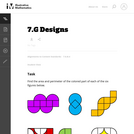Unrestricted Use
CC BY
Rating
0.0 stars

This is a task from the Illustrative Mathematics website that is one part of a complete illustration of the standard to which it is aligned. Each task has at least one solution and some commentary that addresses important asects of the task and its potential use. Here are the first few lines of the commentary for this task: Find the area and perimeter of the colored part of each of the six figures below. The purple, blue, orange, red, and green figures are composed of smal...

Subject:
Mathematics
Material Type:
Activity/Lab
Provider:
Illustrative Mathematics
Provider Set:
Illustrative Mathematics
Author:
Illustrative Mathematics
05/20/2013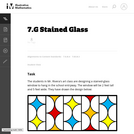Unrestricted Use
CC BY
Rating
0.0 stars

This is a task from the Illustrative Mathematics website that is one part of a complete illustration of the standard to which it is aligned. Each task has at least one solution and some commentary that addresses important asects of the task and its potential use. Here are the first few lines of the commentary for this task: The students in Mr. Rivera's art class are designing a stained-glass window to hang in the school entryway. The window will be 2 feet tall and 5 feet w...

Subject:
Mathematics
Material Type:
Activity/Lab
Provider:
Illustrative Mathematics
Provider Set:
Illustrative Mathematics
Author:
Illustrative Mathematics
08/19/2013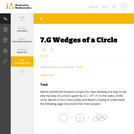Unrestricted Use
CC BY
Rating
0.0 stars

This is a task from the Illustrative Mathematics website that is one part of a complete illustration of the standard to which it is aligned. Each task has at least one solution and some commentary that addresses important aspects of the task and its potential use.

Subject:
Geometry
Mathematics
Material Type:
Activity/Lab
Provider:
Illustrative Mathematics
Provider Set:
Illustrative Mathematics
Author:
Illustrative Mathematics
08/06/2015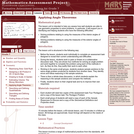Only Sharing Permitted
CC BY-NC-ND
Rating
0.0 stars

This lesson unit is intended to help you assess how well students are able to use geometric properties to solve problems. In particular, it will support you in identifying and helping students who have the following difficulties: Solving problems relating to using the measures of the interior angles of polygons; and solving problems relating to using the measures of the exterior angles of polygons.

Subject:
Geometry
Mathematics
Material Type:
Assessment
Lesson Plan
Provider:
Shell Center for Mathematical Education
Provider Set:
Mathematics Assessment Project (MAP)
04/26/2013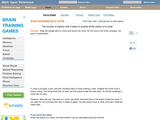Rating
0.0 stars

An interactive applet and associated web page that demonstrate the area of a circle. A circle is shown with a point on the circumference that can be dragged to resize the circle. As the circle is resized, the radius and the area computation is shown changing in real time. The radius and formula can be hidden for class discussion. Applet can be enlarged to full screen size for use with a classroom projector. This resource is a component of the Math Open Reference Interactive Geometry textbook project at http://www.mathopenref.com.

Subject:
Geometry
Mathematics
Material Type:
Simulation
Provider:
Math Open Reference
Author:
John Page
02/16/2011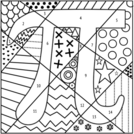Unrestricted Use
CC BY
Rating
0.0 stars

This resource is a 32 slide Desmos Activity. In the activity, students answer questions about calculating the raidus, diameter, circumference and area of given circles. Each correct answer allows them to advance to the Pi Coloring Page to add color to a section.&nbsp;

Subject:
Geometry
Measurement and Data
Material Type:
Activity/Lab
Game
Homework/Assignment
Author:
Sara Scholes
11/18/2020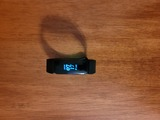Conditional Remix & Share Permitted
CC BY-NC-SA
Rating
0.0 stars

Student discover the relationship between the diameter of a circle and its circumference. Then students practice finding the circumference given the diameter or radius.&nbsp;

Subject:
Mathematics
Material Type:
Lesson
Author:
April Hamma
06/14/2022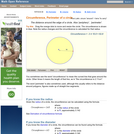Rating
0.0 stars

An interactive applet and associated web page that demonstrate the circumference of a circle. The applet shows a circle with a radius line. The radius endpoints are draggable and the circle is resized accordingly. The formula relating radius to circumference is updated continually as you drag. Introduces the idea of Pi. The formula can be hidden for class discussion and estimation. See also the entries for circumference and diameter. See also entries for radius and diameter. Applet can be enlarged to full screen size for use with a classroom projector. This resource is a component of the Math Open Reference Interactive Geometry textbook project at http://www.mathopenref.com.

Subject:
Geometry
Mathematics
Material Type:
Simulation
Provider:
Math Open Reference
Author:
John Page
02/16/2011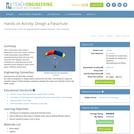Educational Use
Rating
0.0 stars

After a discussion about what a parachute is and how it works, students create parachutes using different materials that they think will work best. They test their designs, and then contribute to a class discussion (and possible journal writing) to report which paper materials worked best.

Subject:
Applied Science
Engineering
Material Type:
Activity/Lab
Provider:
TeachEngineering
Provider Set:
TeachEngineering
09/18/2014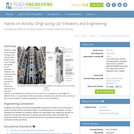Educational Use
Rating
0.0 stars

Students create model elevator carriages and calibrate them, similar to the work of design and quality control engineers. Students use measurements from rotary encoders to recreate the task of calibrating elevators for a high-rise building. They translate the rotations from an encoder to correspond to the heights of different floors in a hypothetical multi-story building. Students also determine the accuracy of their model elevators in getting passengers to their correct destinations.

Subject:
Applied Science
Architecture and Design
Engineering
Material Type:
Activity/Lab
Provider:
TeachEngineering
Provider Set:
TeachEngineering
Author:
Chris Leung
Paul Phamduy
09/18/2014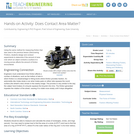Educational Use
Rating
0.0 stars

Using the same method for measuring friction that was used in the previous lesson (Discovering Friction), students design and conduct experiments to determine if the amount of area over which an object contacts a surface it is moving across affects the amount of friction encountered.

Subject:
Applied Science
Engineering
Physical Science
Physics
Material Type:
Activity/Lab
Provider:
TeachEngineering
Provider Set:
TeachEngineering
Author:
Mary R. Hebrank
10/14/2015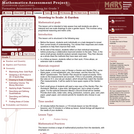Only Sharing Permitted
CC BY-NC-ND
Rating
0.0 stars

This lesson unit is intended to help assess how well students are able to interpret and use scale drawings to plan a garden layout. This involves using proportional reasoning and metric units.

Subject:
Algebra
Geometry
Mathematics
Ratios and Proportions
Material Type:
Assessment
Lesson Plan
Provider:
Shell Center for Mathematical Education
Provider Set:
Mathematics Assessment Project (MAP)
04/26/2013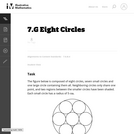Unrestricted Use
CC BY
Rating
0.0 stars

The purpose of this task is to strengthen students' understanding of area. It could be assigned in class to individuals or small groups or given as a homework exercise to generate interesting discussions the following day. The relatively high levels of complexity and technical demand enhance its instructional value.

Subject:
Geometry
Mathematics
Material Type:
Activity/Lab
Provider:
Illustrative Mathematics
Provider Set:
Illustrative Mathematics
Author:
Illustrative Mathematics
05/01/2012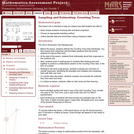Only Sharing Permitted
CC BY-NC-ND
Rating
0.0 stars

This lesson unit is intended to help you assess how well students are able to: solve simple problems involving ratio and direct proportion; choose an appropriate sampling method; and collect discrete data and record them using a frequency table.

Subject:
Education
Geometry
Mathematics
Measurement and Data
Ratios and Proportions
Material Type:
Assessment
Lecture Notes
Lesson Plan
Teaching/Learning Strategy
Provider:
Shell Center for Mathematical Education
Provider Set:
Mathematics Assessment Project (MAP)
Author:
http://map.mathshell.org/
04/26/2013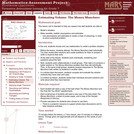Only Sharing Permitted
CC BY-NC-ND
Rating
0.0 stars

This lesson unit is intended to help you assess how well students are able to: Model a situation; make sensible, realistic assumptions and estimates; and use assumptions and estimates to create a chain of reasoning, in order to solve a practical problem.

Subject:
Geometry
Mathematics
Material Type:
Assessment
Lesson Plan
Provider:
Shell Center for Mathematical Education
Provider Set:
Mathematics Assessment Project (MAP)
04/26/2013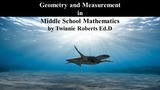Unrestricted Use
CC BY
Rating
0.0 stars

This supplemental resource provides problems and activities related to&nbsp;Geometry and Measurement in Middle School Mathematics.&nbsp;

Subject:
Mathematics
Material Type:
Activity/Lab
Author:
Twianie Roberts Ed.D
09/25/2022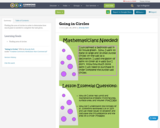Conditional Remix & Share Permitted
CC BY-NC
Rating
0.0 stars

Finding the area of circles in order to determine how much paint is needed to complete the task given.

Subject:
Education
Mathematics
Material Type:
Activity/Lab
01/28/2016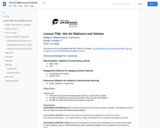Conditional Remix & Share Permitted
CC BY-NC
Rating
0.0 stars

Students will customize a hot air balloon using a non-typical shape and calculate volume

Subject:
Mathematics
Material Type:
Lesson Plan
Provider:
National Air and Space Museum
Author:
National Air and Space Museum
08/30/2022Conditional Remix & Share Permitted
CC BY-NC
Rating
0.0 stars

Four full-year digital course, built from the ground up and fully-aligned to the Common Core State Standards, for 7th grade Mathematics. Created using research-based approaches to teaching and learning, the Open Access Common Core Course for Mathematics&nbsp;is designed with student-centered learning in mind, including activities for students to develop valuable 21st century skills and academic mindset.

Subject:
Mathematics
Material Type:
Full Course
Provider:
Pearson
10/06/2016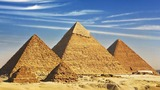Conditional Remix & Share Permitted
CC BY-NC
Rating
0.0 stars

Zooming In On Figures

Unit Overview

Type of Unit: Concept; Project

Length of Unit: 18 days and 5 days for project

Prior Knowledge

Students should be able to:

Find the area of triangles and special quadrilaterals.
Use nets composed of triangles and rectangles in order to find the surface area of solids.
Find the volume of right rectangular prisms.
Solve proportions.

Lesson Flow

After an initial exploratory lesson that gets students thinking in general about geometry and its application in real-world contexts, the unit is divided into two concept development sections: the first focuses on two-dimensional (2-D) figures and measures, and the second looks at three-dimensional (3-D) figures and measures.
The first set of conceptual lessons looks at 2-D figures and area and length calculations. Students explore finding the area of polygons by deconstructing them into known figures. This exploration will lead to looking at regular polygons and deriving a general formula. The general formula for polygons leads to the formula for the area of a circle. Students will also investigate the ratio of circumference to diameter ( pi ). All of this will be applied toward looking at scale and the way that length and area are affected. All the lessons noted above will feature examples of real-world contexts.
The second set of conceptual development lessons focuses on 3-D figures and surface area and volume calculations. Students will revisit nets to arrive at a general formula for finding the surface area of any right prism. Students will extend their knowledge of area of polygons to surface area calculations as well as a general formula for the volume of any right prism. Students will explore the 3-D surface that results from a plane slicing through a rectangular prism or pyramid. Students will also explore 3-D figures composed of cubes, finding the surface area and volume by looking at 3-D views.
The unit ends with a unit examination and project presentations.

Subject:
Geometry
Mathematics
Material Type:
Unit of Study
Provider:
Pearson